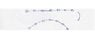# Fitting points from a straight line segment onto a circular segment

• Signifier
Yes, that's correct. But I just want to see what happens if I ignore the position of the carbons and just calculate the force on the atoms as a function of their distance from the center of the circle.

#### Signifier

Hello, I have a problem I need to solve quickly... basically, I have a set of points in 3D space that make up a straight segment of a nanotube, and I want to "bend" the points along a circle (to simulate what happens if you bend a nanotube into a torus). I basically want to do to all of my nanotube segment points what I've indicated in the attached image of points along one straight line.

The problem: I don't know what to do! Is this a relatively simple procedure, or would it be quite a task? Is there a simple operation I can apply to each point (that is say, some distance R from some fixed point and some distance K from some fixed line) that would result in this sort of "central bending" like in the picture?

Any help anyone could provide would be GREATLY, GREATLY appreciated. Thank you!

#### Attachments

•bending.jpg
3.4 KB · Views: 340
What are the forces involved in the bending?

It seems that the points should be connected by hookes law springs. Then it is a matter of solving the diff eq according to the boundary conditions!

I would be happy to help you, using mathematica, if you can better define the goal of the simulation.

Sorry for not giving enough info. I have already written a simulation that connects the points by Hooke's law springs (sort of, there's a few more terms in there); now I just want to wrap the vertices of the nanotube around a circle and show the forces acting on certain atoms that result from this bending. I'm using C++ and OpenGL.

I know what I'm doing is physics simulation faux pas, but I just want to see what will happen. (My simul is not animated, either, it just colors each carbon based on the total force acting on the carbon).

I know what I'm doing is physics simulation faux pas, but I just want to see what will happen. (My simul is not animated, either, it just colors each carbon based on the total force acting on the carbon).

Not a faux pas, just a constraint!

If I understand correctly, you want to setup the position of the carbons to lie on a circle, and then calculate the net force on each carbon?

But doesn't your hooke-like system of equations give you the force on each carbon as a function of its relative position with all others?

## 1. How do you determine the best fit for a straight line onto a circular segment?

The best fit for a straight line onto a circular segment is determined by finding the center and radius of the circle, as well as the slope of the line. This can be done using mathematical equations such as the center-radius form and the slope-intercept form.

## 2. Can you fit more than one straight line onto a circular segment?

Yes, it is possible to fit more than one straight line onto a circular segment. This can be done by dividing the circle into multiple segments and fitting a line onto each segment. Alternatively, a curved line can also be used to fit onto the circular segment.

## 3. What is the purpose of fitting a straight line onto a circular segment?

Fitting a straight line onto a circular segment can be useful in various applications, such as data analysis and graphing. It allows for a more accurate representation of data points that fall onto a circular segment, as opposed to simply connecting the points with straight lines.

## 4. What is the difference between fitting a straight line onto a circular segment and fitting a curve?

The main difference between fitting a straight line onto a circular segment and fitting a curve is the level of accuracy. A straight line only considers the center and radius of the circle, while a curve takes into account the curvature of the circular segment, resulting in a more precise fit.

## 5. Can fitting a straight line onto a circular segment be done manually?

Yes, fitting a straight line onto a circular segment can be done manually by using a ruler and compass to draw the line and measure the center and radius of the circle. However, for more complex calculations and accurate results, it is recommended to use mathematical equations or software programs designed for this purpose.